SLVSB09C September   2011  – October 2017

PRODUCTION DATA.

1. Features
2. Applications
3. Description
4. Revision History
5. Pin Configuration and Functions
6. Specifications
7. Detailed Description
1. 7.1 Overview
2. 7.2 Functional Block Diagram
3. 7.3 Feature Description
4. 7.4 Device Functional Modes
8. Application and Implementation
1. 8.1 Application Information
2. 8.2 Typical Application
1. 8.2.1 Design Requirements
2. 8.2.2 Detailed Design Procedure
3. 8.2.3 Application Curves
9. Power Supply Recommendations
10. 10Layout
1. 10.1 Layout Guidelines
2. 10.2 Layout Examples
11. 11Device and Documentation Support
12. 12Mechanical, Packaging, and Orderable Information

• RHL|14

## 7 Detailed Description

### 7.1 Overview

The device is a 17-V, 6-A, synchronous step-down (buck) converter with two integrated n-channel MOSFETs. To improve performance during line and load transients the device implements a constant frequency, peak current mode control which also simplifies external frequency compensation. The wide switching frequency of 200 kHz to 1600 kHz allows for efficiency and size optimization when selecting the output filter components. The switching frequency is adjusted using a resistor to ground on the RT/CLK pin. The device also has an internal phase lock loop (PLL) controlled by the RT/CLK pin that can be used to synchronize the switching cycle to the falling edge of an external system clock.

The device has been designed for safe monotonic start-up into pre-biased loads. The default start up is when VIN is typically 4 V. The EN pin has an internal pullup current source that can be used to adjust the input voltage under voltage lockout (UVLO) with two external resistors. In addition, the EN pin can be floating for the device to operate with the internal pullup current. The total operating current for the device is approximately 600 μA when not switching and under no load. When the device is disabled, the supply current is typically less than 2 μA.

The integrated MOSFETs allow for high efficiency power supply designs with continuous output currents up to 6 A. The MOSFETs have been sized to optimize efficiency for lower duty cycle applications.

The device reduces the external component count by integrating the boot recharge circuit. The bias voltage for the integrated high-side MOSFET is supplied by a capacitor between the BOOT and PH pins. The boot capacitor voltage is monitored by a BOOT to PH UVLO (BOOT-PH UVLO) circuit allowing PH pin to be pulled low to recharge the boot capacitor. The device can operate at 100% duty cycle as long as the boot capacitor voltage is higher than the preset BOOT-PH UVLO threshold which is typically 2.1 V. The output voltage can be stepped down to as low as the 0.6 V voltage reference (Vref).

The device has a power good comparator (PWRGD) with hysteresis which monitors the output voltage through the VSENSE pin. The PWRGD pin is an open drain MOSFET which is pulled low when the VSENSE pin voltage is less than 92% or greater than 106% of the reference voltage Vref and asserts high when the VSENSE pin voltage is 94% to 104% of the Vref.

The SS/TR (slow start/tracking) pin is used to minimize inrush currents or provide power supply sequencing during power up. A small value capacitor or resistor divider should be coupled to the pin for slow start or critical power supply sequencing requirements.

The device is protected from output overvoltage, overload and thermal fault conditions. The device minimizes excessive output overvoltage transients by taking advantage of the overvoltage circuit power good comparator. When the overvoltage comparator is activated, the high-side MOSFET is turned off and prevented from turning on until the VSENSE pin voltage is lower than 104% of the Vref. The device implements both high-side MOSFET overload protection and bidirectional low-side MOSFET overload protections which help control the inductor current and avoid current runaway. The device also shuts down if the junction temperature is higher than thermal shutdown trip point. The device is restarted under control of the slow start circuit automatically after the built-in thermal shutdown hiccup time.

The TPS54623 monitors the peak switch current of the high-side MOSFET. Once the peak switch current is lower than typically 1 A, the device stops switching to boost the efficiency until the peak switch current is higher than typically 1 A again.

### 7.2 Functional Block Diagram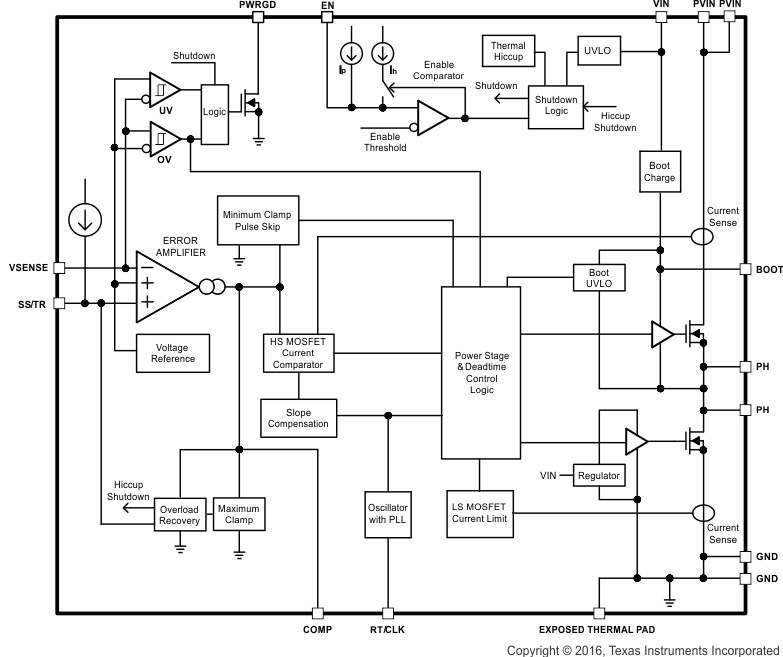### 7.3 Feature Description

#### 7.3.1 VIN and Power VIN Pins (VIN and PVIN)

The device allows for a variety of applications by using the VIN and PVIN pins together or separately. The VIN pin voltage supplies the internal control circuits of the device. The PVIN pin voltage provides the input voltage to the power converter system.

If tied together, the input voltage for VIN and PVIN can range from 4.5 V to 17 V. If using the VIN separately from PVIN, the VIN pin must be between 4.5 V and 17 V, and the PVIN pin can range from as low as 1.6 V to 17 V. A voltage divider connected to the EN pin can adjust the either input voltage UVLO appropriately. Adjusting the input voltage UVLO on the PVIN pin helps to provide consistent power up behavior.

#### 7.3.2 Voltage Reference

The voltage reference system produces a precise ±1% voltage reference over temperature by scaling the output of a temperature stable bandgap circuit.

#### 7.3.3 Adjusting the Output Voltage

The output voltage is set with a resistor divider from the output (VOUT) to the VSENSE pin. It is recommended to use 1% tolerance or better divider resistors. Referring to the application schematic of Figure 31, start with a 10 kΩ for R6 and use Equation 1 to calculate R5. To improve efficiency at light loads consider using larger value resistors. If the values are too high the regulator is more susceptible to noise and voltage errors from the VSENSE input current are noticeable.

Equation 1.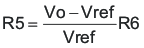where

• Vref = 0.6 V

The minimum output voltage and maximum output voltage can be limited by the minimum on time of the high-side MOSFET and bootstrap voltage (BOOT-PH voltage) respectively. More discussions are located in Minimum Output Voltage and Bootstrap Voltage (BOOT) and Low Dropout Operation.

#### 7.3.4 Safe Start-up into Pre-Biased Outputs

The device has been designed to prevent the low-side MOSFET from discharging a pre-biased output. During monotonic pre-biased startup, the low-side MOSFET is not allowed to sink current until the SS/TR pin voltage is higher than 1.4 V.

#### 7.3.5 Error Amplifier

The device uses a transconductance error amplifier. The error amplifier compares the VSENSE pin voltage to the lower of the SS/TR pin voltage or the internal 0.6 V voltage reference. The transconductance of the error amplifier is 1300 μA/V during normal operation. The frequency compensation network is connected between the COMP pin and ground.

#### 7.3.6 Slope Compensation

The device adds a compensating ramp to the switch current signal. This slope compensation prevents sub-harmonic oscillations. The available peak inductor current remains constant over the full duty cycle range.

#### 7.3.7 Enable and Adjusting Undervoltage Lockout

The EN pin provides electrical on/off control of the device. Once the EN pin voltage exceeds the threshold voltage, the device starts operation. If the EN pin voltage is pulled below the threshold voltage, the regulator stops switching and enters low Iq state.

The EN pin has an internal pullup current source, allowing the user to float the EN pin for enabling the device. If an application requires controlling the EN pin, use open drain or open collector output logic to interface with the pin.

The device implements internal UVLO circuitry on the VIN pin. The device is disabled when the VIN pin voltage falls below the internal VIN UVLO threshold. The internal VIN UVLO threshold has a hysteresis of 150 mV.

If an application requires either a higher UVLO threshold on the VIN pin or a secondary UVLO on the PVIN, in split rail applications, then the EN pin can be configured as shown in Figure 17, Figure 18 and Figure 19. When using the external UVLO function it is recommended to set the hysteresis to be greater than 500 mV.

The EN pin has a small pullup current Ip which sets the default state of the pin to enable when no external components are connected. The pullup current is also used to control the voltage hysteresis for the UVLO function since it increases by Ih once the EN pin crosses the enable threshold. The UVLO thresholds can be calculated using Equation 2 and Equation 3.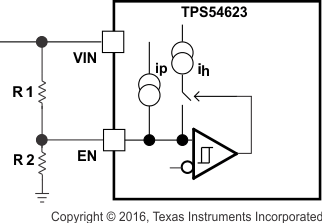Figure 17. Adjustable VIN Undervoltage Lockout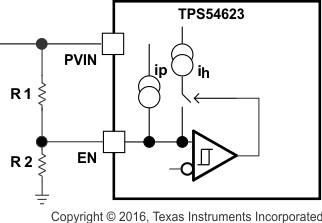Figure 18. Adjustable PVIN Undervoltage Lockout, VIN ≥ 4.5 V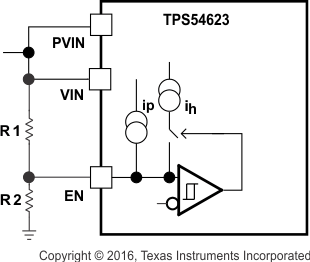Figure 19. Adjustable VIN and PVIN Undervoltage Lockout
Equation 2.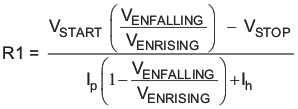Equation 3.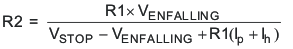where

• Ih = 3.4 μA
• Ip = 1.15 μA
• VENRISING = 1.21 V
• VENFALLING = 1.17 V

#### 7.3.8 Slow Start (SS/TR)

The device uses the lower voltage of the internal voltage reference or the SS/TR pin voltage as the reference voltage and regulates the output accordingly. A capacitor on the SS/TR pin to ground implements a slow start time. The device has an internal pullup current source of 2.3 μA that charges the external slow start capacitor. The calculations for the slow start time (Tss, 10% to 90%) and slow start capacitor (Css) are shown in Equation 4. The voltage reference (Vref) is 0.6 V and the slow start charge current (Iss) is 2.3 μA.

Equation 4.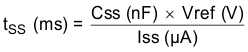When the input UVLO is triggered, the EN pin is pulled below 1.21 V, or a thermal shutdown event occurs the device stops switching and enters low current operation. At the subsequent power up, when the shutdown condition is removed, the device does not start switching until it has discharged its SS/TR pin to ground ensuring proper soft start behavior.

#### 7.3.9 Power Good (PWRGD)

The PWRGD pin is an open drain output. Once the VSENSE pin is between 94% and 104% of the internal voltage reference the PWRGD pin pull-down is de-asserted and the pin floats. It is recommended to use a pullup resistor between the values of 10 kΩ and 100 kΩ to a voltage source that is 5.5 V or less. The PWRGD is in a defined state once the VIN input voltage is greater than 1 V but with reduced current sinking capability. The PWRGD achieves full current sinking capability once the VIN input voltage is above 4.5 V.

The PWRGD pin is pulled low when VSENSE is lower than 92% or greater than 106% of the nominal internal reference voltage. Also, the PWRGD is pulled low, if the input UVLO or thermal shutdown are asserted, the EN pin is pulled low or the SS/TR pin is below 1.4 V.

#### 7.3.10 Bootstrap Voltage (BOOT) and Low Dropout Operation

The device has an integrated boot regulator, and requires a small ceramic capacitor between the BOOT and PH pins to provide the gate drive voltage for the high-side MOSFET. The boot capacitor is charged when the BOOT pin voltage is less than VIN and BOOT-PH voltage is below regulation. The value of this ceramic capacitor should be 0.1 μF. A ceramic capacitor with an X7R or X5R grade dielectric with a voltage rating of 10 V or higher is recommended because of the stable characteristics over temperature and voltage.

To improve drop out, the device is designed to operate at 100% duty cycle as long as the BOOT to PH pin voltage is greater than the BOOT-PH UVLO threshold which is typically 2.1 V. When the voltage between BOOT and PH drops below the BOOT-PH UVLO threshold the high-side MOSFET is turned off and the low-side MOSFET is turned on allowing the boot capacitor to be recharged. In applications with split input voltage rails 100% duty cycle operation can be achieved as long as (VIN – PVIN) > 4 V.

#### 7.3.11 Sequencing (SS/TR)

Many of the common power supply sequencing methods can be implemented using the SS/TR, EN and PWRGD pins.

The sequential method is illustrated in Figure 20 using two TPS54623 devices. The power good of the first device is coupled to the EN pin of the second device, which enables the second power supply once the primary supply reaches regulation.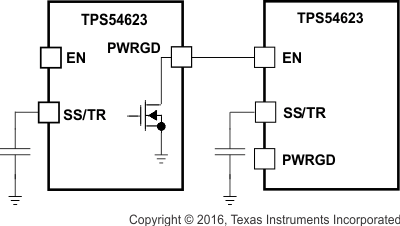Figure 20. Sequential Start-Up Sequence

Figure 21 shows the method implementing ratio-metric sequencing by connecting the SS/TR pins of two devices together. The regulator outputs ramp up and reach regulation at the same time. When calculating the slow start time the pullup current source must be doubled in Equation 4.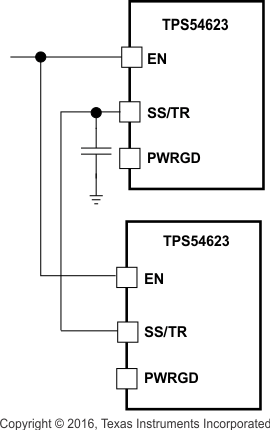Figure 21. Ratiometric Start-Up Sequence

Ratio-metric and simultaneous power supply sequencing can be implemented by connecting the resistor network of R1 and R2 shown in Figure 22 to the output of the power supply that needs to be tracked or another voltage reference source. Using Equation 5 and Equation 6, the tracking resistors can be calculated to initiate the Vout2 slightly before, after or at the same time as Vout1. Equation 7 is the voltage difference between Vout1 and Vout2.

To design a ratio-metric start up in which the Vout2 voltage is slightly greater than the Vout1 voltage when Vout2 reaches regulation, use a negative number in Equation 5 and Equation 6 for deltaV. Equation 7 results in a positive number for applications where the Vout2 is slightly lower than Vout1 when Vout2 regulation is achieved. .

The deltaV variable is zero volt for simultaneous sequencing. To minimize the effect of the inherent SS/TR to VSENSE offset (Vssoffset, 29 xmV) in the slow start circuit and the offset created by the pullup current source (Iss, 2.3 μA) and tracking resistors, the Vssoffset and Iss are included as variables in the equations.

To ensure proper operation of the device, the calculated R1 value from Equation 5 must be greater than the value calculated in Equation 8.

Equation 5.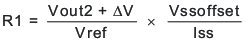Equation 6.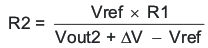Equation 7.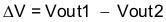Equation 8.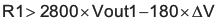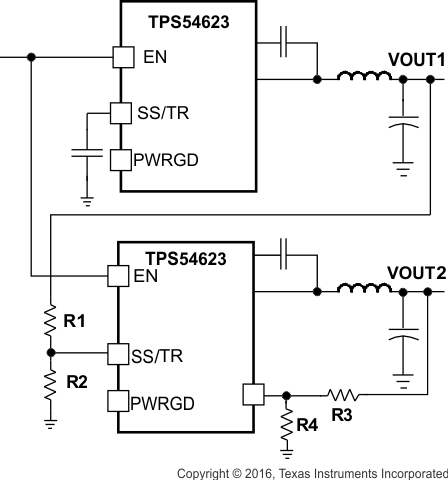Figure 22. Ratiometric and Simultaneous Start-up Sequence

There are two final considerations when using a resistor divider to the SS/TR pin for simultaneous start-up. First, as described in Power Good (PWRGD), for the PWRGD output to be active the SS/TR voltage must be above 1.4 V. The external divider may prevent the SS/TR voltage from charging above the threshold. For the SS/TR pin to charge above the threshold, an external MOSFET may be needed to disconnect the resistor divider or modify the resistor divider ratio after start-up is complete. The PWRGD pin of the VOUT(1) converter could be used to turn on or turn off the external MOSFET. Second, a pre-bias on VOUT(1) may prevent VOUT(2) from turning on. When the TPS54623 is enabled, an internal 700-Ω MOSFET at the SS/TR pin turns on to discharge the SS/TR voltage as described in Slow Start (SS/TR). The SS/TR pin voltage must discharge below 20 mV before the TPS54623 starts up. If the upper resistor at the SS/TR pin is too small, the SS/TR pin does not discharge below the threshold, and VOUT(2) does not ramp up. The upper resistor in the SS/TR divider may need to be increased to allow the SS/TR pin to discharge below the threshold.

#### 7.3.12 Output Overvoltage Protection (OVP)

The device incorporates an output overvoltage protection (OVP) circuit to minimize output voltage overshoot. For example, when the power supply output is overloaded the error amplifier compares the actual output voltage to the internal reference voltage. If the VSENSE pin voltage is lower than the internal reference voltage for a considerable time, the output of the error amplifier demands maximum output current. Once the condition is removed, the regulator output rises and the error amplifier output transitions to the steady state voltage. In some applications with small output capacitance, the power supply output voltage can respond faster than the error amplifier. This leads to the possibility of an output overshoot. The OVP feature minimizes the overshoot by comparing the VSENSE pin voltage to the OVP threshold. If the VSENSE pin voltage is greater than the OVP threshold the high-side MOSFET is turned off preventing current from flowing to the output and minimizing output overshoot. When the VSENSE voltage drops lower than the OVP threshold, the high-side MOSFET is allowed to turn on at the next clock cycle.

#### 7.3.13 Overcurrent Protection

The device is protected from overcurrent conditions by cycle-by-cycle current limiting on both the high-side MOSFET and the low-side MOSFET.

#### 7.3.13.1 High-side MOSFET Overcurrent Protection

The device implements current mode control which uses the COMP pin voltage to control the turnoff of the high-side MOSFET and the turnon of the low-side MOSFET on a cycle by cycle basis. Each cycle the switch current and the current reference generated by the COMP pin voltage are compared, when the peak switch current intersects the current reference the high-side switch is turned off.

#### 7.3.13.2 Low-side MOSFET Overcurrent Protection

While the low-side MOSFET is turned on its conduction current is monitored by the internal circuitry. During normal operation the low-side MOSFET sources current to the load. At the end of every clock cycle, the low-side MOSFET sourcing current is compared to the internally set low-side sourcing current limit. If the low-side sourcing current is exceeded the high-side MOSFET is not turned on and the low-side MOSFET stays on for the next cycle. The high-side MOSFET is turned on again when the low-side current is below the low-side sourcing current limit at the start of a cycle.

The low-side MOSFET may also sink current from the load. If the low-side sinking current limit is exceeded the low-side MOSFET is turned off immediately for the rest of that clock cycle. In this scenario both MOSFETs are off until the start of the next cycle.

Furthermore, if an output overload condition (as measured by the COMP pin voltage) has lasted for more than the hiccup wait time which is programmed for 512 switching cycles, the device shuts down itself and restart after the hiccup time of 16384 cycles. The hiccup mode helps to reduce the device power dissipation under severe overcurrent conditions.

#### 7.3.14 Thermal Shutdown

The internal thermal shutdown circuitry forces the device to stop switching if the junction temperature exceeds 175°C typically. Once the junction temperature drops below 165°C typically, the internal thermal hiccup timer will start to count. The device reinitiates the power up sequence after the built-in thermal shutdown hiccup time (16384 cycles) is over.

#### 7.3.15 Small Signal Model for Loop Response

Figure 23 shows an equivalent model for the device control loop which can be modeled in a circuit simulation program to check frequency response and transient responses. The error amplifier is a transconductance amplifier with a gm of 1300μA/V. The error amplifier can be modeled using an ideal voltage controlled current source. The resistor Roea (2.38 MΩ) and capacitor Coea (20.7 pF) model the open loop gain and frequency response of the error amplifier. The 1-mV ac voltage source between the nodes a and b effectively breaks the control loop for the frequency response measurements. Plotting a/c and c/b show the small signal responses of the power stage and frequency compensation respectively. Plotting a/b shows the small signal response of the overall loop. The dynamic loop response can be checked by replacing the RL with a current source with the appropriate load step amplitude and step rate in a time domain analysis.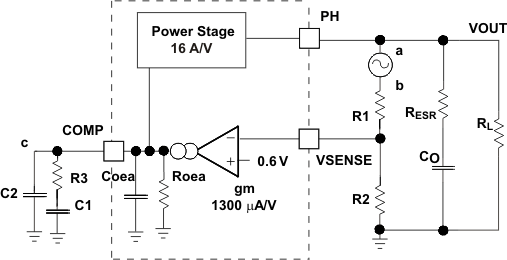Figure 23. Small Signal Model for Loop Response

#### 7.3.16 Simple Small Signal Model for Peak Current Mode Control

Figure 24 is a simple small signal model that can be used to understand how to design the frequency compensation. The device power stage can be approximated to a voltage controlled current source (duty cycle modulator) supplying current to the output capacitor and load resistor. The control to output transfer function is shown in Equation 9 and consists of a dc gain, one dominant pole and one ESR zero. The quotient of the change in switch current and the change in COMP pin voltage (node c in Figure 23) is the power stage transconductance (gmps) which is 16 A/V for the device. The DC gain of the power stage is the product of gmps and the load resistance RL) as shown in Equation 10 with resistive loads. As the load current increases, the DC gain decreases. This variation with load may seem problematic at first glance, but fortunately the dominant pole moves with load current (see Equation 11). The combined effect is highlighted by the dashed line in Figure 25. As the load current decreases, the gain increases and the pole frequency lowers, keeping the 0-dB crossover frequency the same for the varying load conditions which makes it easier to design the frequency compensation.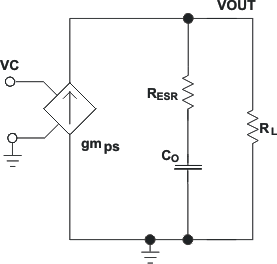Figure 24. Simplified Small Signal Model for Peak Current Mode Control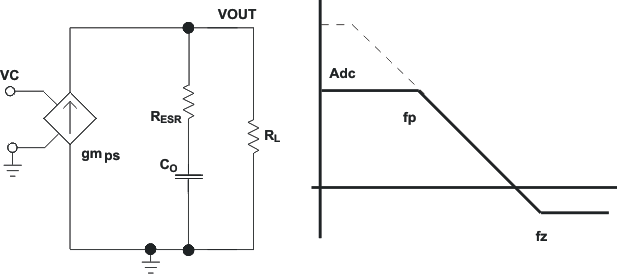Figure 25. Simplified Frequency Response for Peak Current Mode Control
Equation 9.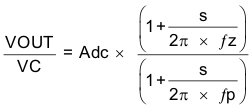Equation 10.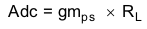Equation 11.Equation 12.where

• gmea is the GM amplifier gain (1300 μA/V)
• gmps is the power stage gain (16 A/V)
• RL is the load resistance
• CO is the output capacitance
• RESR is the equivalent series resistance of the output capacitor

#### 7.3.17 Small Signal Model for Frequency Compensation

The device uses a transconductance amplifier for the error amplifier and readily supports two of the commonly used Type II compensation circuits and a Type III frequency compensation circuit, as shown in Figure 26. In Type 2A, one additional high frequency pole, C6, is added to attenuate high frequency noise. In Type III, one additional capacitor, C11, is added to provide a phase boost at the crossover frequency. See Designing Type III Compensation for Current Mode Step-Down Converters (for a complete explanation of Type III compensation.

The design guidelines below are provided for advanced users who prefer to compensate using the general method. The below equations only apply to designs whose ESR zero is above the bandwidth of the control loop. This is usually true with ceramic output capacitors. See Typical Application for a step-by-step design procedure using higher ESR output capacitors with lower ESR zero frequencies.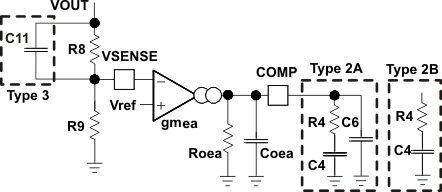Figure 26. Types of Frequency Compensation

The general design guidelines for device loop compensation are as follows:

1. Determine the crossover frequency, fc. A good starting point is 1/10th of the switching frequency, fsw.
2. R4 can be determined by Equation 13.
3. Equation 13.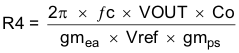where

• gmea is the GM amplifier gain (1300 μA/V)
• gmps is the power stage gain (16 A/V)
• Vref is the reference voltage (0.6 V)
4. Place a compensation zero at the dominant pole: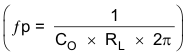C4 can be determined by Equation 14.
5. Equation 14.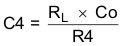6. C6 is optional. It can be used to cancel the zero from the equivalent series resistance (ESR) of the output capacitor Co.
7. Equation 15.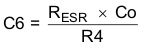8. Type III compensation can be implemented with the addition of one capacitor, C11. This allows for slightly higher loop bandwidths and higher phase margins. If used, C11 is calculated from Equation 16.
9. Equation 16.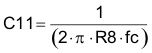### 7.4 Device Functional Modes

#### 7.4.1 Fixed Frequency PWM Control

The device uses a adjustable fixed frequency, peak current mode control. The output voltage is compared through external resistors on the VSENSE pin to an internal voltage reference by an error amplifier which drives the COMP pin. An internal oscillator initiates the turn on of the high-side power switch. The error amplifier output is converted into a current reference which compares to the high-side power switch current. When the power switch current reaches current reference generated by the COMP voltage level the high-side power switch is turned off and the low-side power switch is turned on.

#### 7.4.2 Continuous Current Mode Operation (CCM)

As a synchronous buck converter, the device normally works in CCM (Continuous Conduction Mode) under load conditions where the minimum inductor valley current is higher than 0 A.

#### 7.4.3 Light Load Efficiency Operation

The TPS54623 operates in pulse skip mode (see Figure 28) at light load currents to improve efficiency by reducing switching and gate drive losses. The TPS54623 is designed so that if the output voltage is within regulation and the peak switch current at the end of any switching cycle is below the pulse skipping current threshold, the device enters pulse skip mode. This current threshold is the current level corresponding to a nominal COMP voltage of 250 mV.

When in pulse skip mode, the COMP pin voltage is clamped and the high side MOSFET is inhibited. Further decreases in load current or in output voltage can not drive the COMP pin below this clamp voltage level.

Since the device is not switching, the output voltage begins to decay. As the voltage control loop compensates for the falling output voltage, the COMP pin voltage begins to rise. At this time, the high side MOSFET is enabled and a switching pulse initiates on the next switching cycle. The peak current is set by the COMP pin voltage. The output voltage re-charges the regulated value, then the peak switch current starts to decrease, and eventually falls below the pulse skip mode threshold at which time the device again enters pulse skip mode.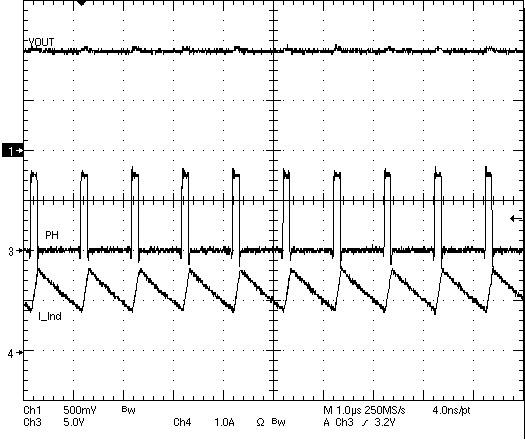Figure 27. TPS54623 Operation In Current Mode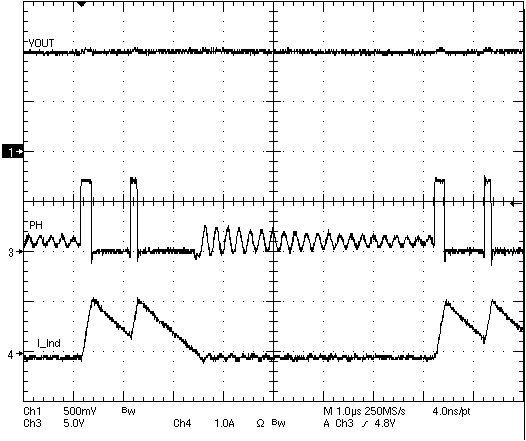Figure 28. TPS54623 Operation In Pulse Skipping Mode

#### 7.4.4 Adjustable Switching Frequency and Synchronization (RT/CLK)

The RT/CLK pin can be used to set the switching frequency of the device in two modes.

In RT mode, a resistor (RT resistor) is connected between the RT/CLK pin and GND. The switching frequency of the device is adjustable from 200 kHz to 1600 kHz by placing a maximum of 240 kΩ and minimum of 29 kΩ respectively. In CLK mode, an external clock is connected directly to the RT/CLK pin. The device is synchronized to the external clock frequency with PLL.

The CLK mode overrides the RT mode. The device is able to detect the proper mode automatically and switch from the RT mode to CLK mode.

#### 7.4.4.1 Adjustable Switching Frequency (RT Mode)

To determine the RT resistance for a given switching frequency, use Equation 17 or the curve in Figure 29. To reduce the solution size one would set the switching frequency as high as possible, but tradeoffs of the supply efficiency and minimum controllable on time should be considered.

Equation 17.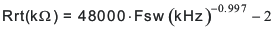Figure 29. RT Set Resistor vs Switching Frequency

#### 7.4.4.2 Synchronization (CLK mode)

An internal phase locked loop (PLL) has been implemented to allow synchronization between 200 kHz and 1600 kHz, and to easily switch from RT mode to CLK mode.

To implement the synchronization feature, connect a square wave clock signal to the RT/CLK pin with a duty cycle between 20% to 80%. The clock signal amplitude must transition lower than 0.8 V and higher than 2 V. The start of the switching cycle is synchronized to the falling edge of RT/CLK pin.

In applications where both RT mode and CLK mode are needed, the device can be configured as shown in Figure 30. Before the external clock is present, the device works in RT mode and the switching frequency is set by RT resistor. When the external clock is present, the CLK mode overrides the RT mode. The first time the SYNC pin is pulled above the RT/CLK high threshold (2 V), the device switches from the RT mode to the CLK mode and the RT/CLK pin becomes high impedance as the PLL starts to lock onto the frequency of the external clock. It is not recommended to switch from the CLK mode back to the RT mode because the internal switching frequency drops to 100 kHz first before returning to the switching frequency set by RT resistor.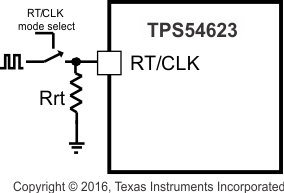Figure 30. Works with Both RT Mode and CLK Mode